Courses

# Chapter 5 Fluid Dynamics - Fluid Mechanics, Mechanical Engineering Mechanical Engineering Notes | EduRev

## Mechanical Engineering : Chapter 5 Fluid Dynamics - Fluid Mechanics, Mechanical Engineering Mechanical Engineering Notes | EduRev

The document Chapter 5 Fluid Dynamics - Fluid Mechanics, Mechanical Engineering Mechanical Engineering Notes | EduRev is a part of the Mechanical Engineering Course Mechanical Engineering SSC JE (Technical).
All you need of Mechanical Engineering at this link: Mechanical Engineering

FLUID DYNAMICS

• Reynolds equation  = Intertia force + gravity force + viscous force + turbulence force + pressure force 
• Navier - Stoke's equation = Intertia gravity force  +  pressure force  +  viscous force 
• Eulers equation (represents momentum equation in a 2-D, inviscid steady flow) Inertia force = gravity force  +  pressure force 
• Bernoulli's equation (Conservation of Energy)
Assumptions in Bernoullis equations:
(i) fluid is ideal
(iii) flow is continous
(iv) fluid is incompressible
(v) flow is non-viscous
(vi) flow is irrotational
(vii) applicable along a stream line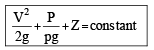where,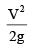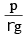z = elevation of datum head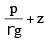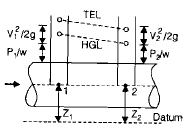• The line representing the sum of all 3 heads is known as total energy line or total head line. 
• Line joining the points of piezometric heads is known as hydraulic grade line or piezometric line. 
• Piezometric head remains constant normal to the stream lines in case of uniform diameter straight pipe. 
• Flow in pipe bend, considered as irrotational flow. Piezometric head line for outer boundary is above than the inner boundary and pressure is also more at outer boundary 
• HGL is always parallel and lower than TEL.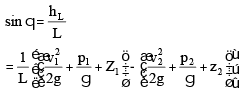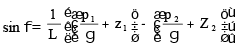• Kinetic Energy correction factor
(i) For laminar flow in pipes, a = 2
(ii) For fully develop turbulent flow in pipes, a = 1.33 Lower value is applicable for rough surface and high Reynolds number. 
• Pressure at stagnation point where velocity of flow is zero is known as the stagnation pressure.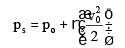where p= static pressure and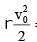= dynamic pressure

•  Flow through Pipe bend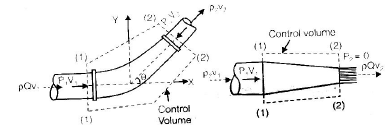P1A1 – P2A2 cos q +
Fx = rQ (V2 cos q – V1)
Fy – P2A2 sin q = rQ (V2 sin q – 0)
Fx and Fy represents the reaction of bend on water. 

• Torque exerted by the water on the pipe will be
T = rQ1 V1 r1 - rQ2 V2 r2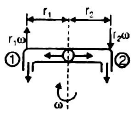V1 = tangential velocity component of absolute velocity at 1
V2 = tangential velocity component of absolute velocity at 2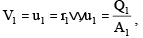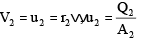Offer running on EduRev: Apply code STAYHOME200 to get INR 200 off on our premium plan EduRev Infinity!

83 docs|53 tests

,

,

,

,

,

,

,

,

,

,

,

,

,

,

,

,

,

,

,

,

,

,

,

,

;